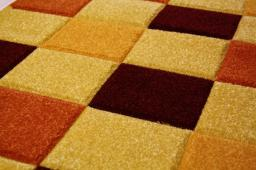# Dimensions 4118

The width of the rectangle is 17 cm smaller than its length. The perimeter of the rectangle measures 0.9 m. Find the remaining dimensions and calculate their area.

a =  31 cm
b =  14 cm
S =  434 cm2

### Step-by-step explanation:

a=17+b
2(a+b)=90

a=17+b
2·(a+b)=90

a-b = 17
2a+2b = 90

Pivot: Row 1 ↔ Row 2
2a+2b = 90
a-b = 17

Row 2 - 1/2 · Row 1 → Row 2
2a+2b = 90
-2b = -28

b = -28/-2 = 14
a = 90-2b/2 = 90-2 · 14/2 = 31

a = 31
b = 14

Our linear equations calculator calculates it.Did you find an error or inaccuracy? Feel free to write us. Thank you!

Tips for related online calculators
Do you have a linear equation or system of equations and looking for its solution? Or do you have a quadratic equation?
Do you want to convert length units?

#### Grade of the word problem:

We encourage you to watch this tutorial video on this math problem: